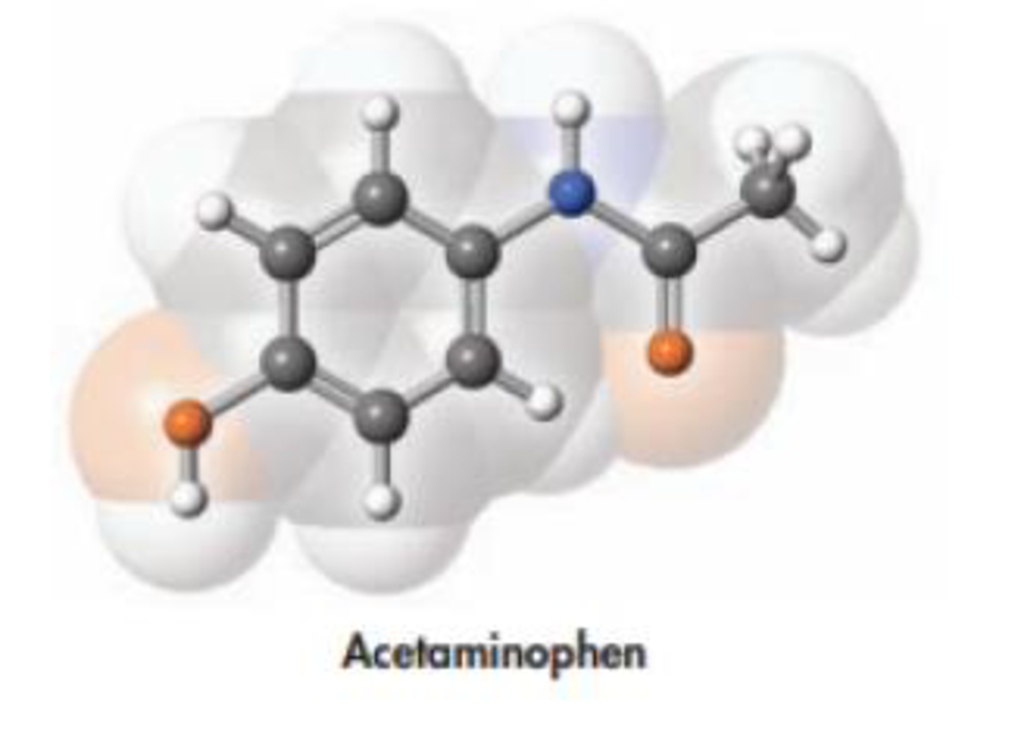# Acetaminophen, whose structure is drawn below, is the active ingredient in some nonprescription pain killers. The recommended dose for an adult is two 500-mg caplets. How many molecules make up one dose of this drug?### Chemistry & Chemical Reactivity

9th Edition
John C. Kotz + 3 others
Publisher: Cengage Learning
ISBN: 9781133949640

#### Solutions

Chapter
Section### Chemistry & Chemical Reactivity

9th Edition
John C. Kotz + 3 others
Publisher: Cengage Learning
ISBN: 9781133949640
Chapter 2, Problem 77PS
Textbook Problem
132 views

## Acetaminophen, whose structure is drawn below, is the active ingredient in some nonprescription pain killers. The recommended dose for an adult is two 500-mg caplets. How many molecules make up one dose of this drug?Interpretation Introduction

Interpretation: The number of molecules present in two 500mg  caplets is to be calculated.

Concept introduction:

• Molar mass of a molecule can be calculated from its molecular formula by taking the sum of atomic masses of all the elements present in it.
• Conversion formula for mass of a molecule into number moles,

Numberofmoles=MassingramsMolarmass

• Equation for finding number of molecules from number of moles of a molecule,

Numberofmolecule=numberofmolesofmolecule×6.022×1023molecules/mol

### Explanation of Solution

The amount of two 500mg acetaminophen caplets is 1000mg.

The molar mass of acetaminophen is 151.163g.

Conversion formula for mass of a molecule into number moles,

Numberofmoles=MassingramsMolarmass

Therefore,

The number moles of acetaminophen is,

Numberofmoles=1000g151.165g/mol=6

### Still sussing out bartleby?

Check out a sample textbook solution.

See a sample solution

#### The Solution to Your Study Problems

Bartleby provides explanations to thousands of textbook problems written by our experts, many with advanced degrees!

Get Started

Find more solutions based on key concepts
Name the following compounds: SO3 HNO2 Mg3N3 HI(aq) Cu3(PO4)2 CuSO45H2O

General Chemistry - Standalone book (MindTap Course List)

The polysaccharide that helps form the supporting structures of plants is cellulose maltose glycogen sucrose

Nutrition: Concepts and Controversies - Standalone book (MindTap Course List)

What is the usefulness of being camouflaged in natural environments?

Biology: The Dynamic Science (MindTap Course List)

Why is degenerate matter so difficult to compress?

Horizons: Exploring the Universe (MindTap Course List)

A parallel-plate capacitor filled with air carries a charge Q. The batten is disconnected, and a slab of materi...

Physics for Scientists and Engineers, Technology Update (No access codes included)